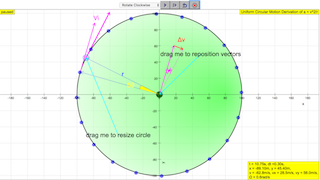### Translations

Code Language Translator Run### Software Requirements

SoftwareRequirements

 Android iOS Windows MacOS with best with Chrome Chrome Chrome Chrome support full-screen? Yes. Chrome/Opera No. Firefox/ Samsung Internet Not yet Yes Yes cannot work on some mobile browser that don't understand JavaScript such as..... cannot work on Internet Explorer 9 and below

### CreditsFu-Kwun Hwang and lookang; lookang; tinatan

### end faq

http://iwant2study.org/lookangejss/02_newtonianmechanics_6circle/ejss_model_circularmotionwee02/circularmotionwee02_Simulation.xhtml

[text]

### For Teachers

A particle is under acceleration when it's speed changed over time. When a particle is moving around a circle with constant speed.
The velocity is changed constantly. The direction of the velocity vector is changing with time. (Velocity is a vector.)

You can click Play to start the simulation and click pause to stop it.
When the simulation is paused at time t: The RED arrow shows the velocity vector at that time.
Another vector in Magenta shows its velocity at previous time step t-dt.
(The starting point for the above two vector is different)

We also draw those two vectors again from the same starting point (which is the center of the circle).
You can find out the difference between two vectors (GREEN vector).

Click STEP button to make the time step forward and watch the differences.

Magenta colour arrow is the instance velocity $$v_{i}(t)$$, where subscript i is for initial
arrow with color magenta after some time dt, is $$v_{f}(t+dt)$$ where subscript f is for final
The red arrow is $$d\vec{v}=\vec{v_{f}}(t+dt)-\vec{_{i}v}(t)$$ which is always pointing toward the center.
with  $$d\theta=v*dt/R$$ ,
so It's length $$|d\vec{v}|=v d\theta= \frac{ (v)(v)dt}{R}$$,
so $$\frac{d\vec{v}}{dt}=\frac{v^2}{R}$$

[text]

[text]

### Other Resources

https://ggbm.at/rjza5pwy by tan send kwang

# Testimonials (0)

There are no testimonials available for viewing. Login to deploy the article and be the first to submit your review!